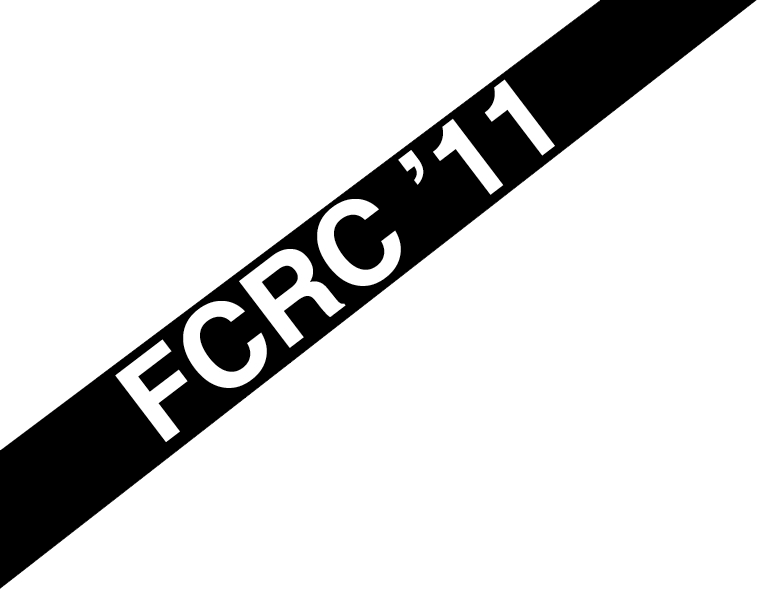# Tutorials

Three tutorials will be held on Wednesday, June 8.Bürgisser

 Peter Bürgisser University of Paderborn, Germany.

## Probabilistic Analysis of Condition Numbers

4.10PM - 6.30PM (new schedule)

Condition numbers are well known in numerical linear algebra. It is less known that this concept also plays a crucial part in understanding the efficiency of algorithms in linear programming, convex optimization, and for solving systems of polynomial equations. Indeed, the running time of such algorithms may be often effectively bounded in terms of the condition underlying the problem.

“Smoothed analysis”, as suggested by Spielman and Teng, is a blend of worst-case and average-case probabilistic analysis of algorithms. The goal is to prove that for all inputs (even ill-posed ones), and all slight random perturbations of that input, it is unlikely that the running time (or condition number) will be large.

The probabilistic analysis of condition numbers can be often rephrased as a geometric problem. It is naturally related to questions concerning the volume of (local) tubes around a subvariety of ill-posed inputs.

The tutorial will present a unifying view on the notion of condition in linear algebra, convex optimization, and polynomial equations. We will discuss the role of condition for the analysis of algorithms as well as techniques for their probabilistic analysis.Kauers

 Manuel Kauers, Research Institute of Symbolic Computation, Johannes Kepler Universität, Austria.

## The Concrete Tetrahedron

1.30PM - 3.50PM (new schedule)

Questions arising in discrete mathematics tend to require calculations involving symbolic sums, recurrence equations, generating functions, and asymptotic estimates. These four mathematical concepts do not stand for their own but rather form the four corners of a compound which we call the concrete tetrahedron. We will survey the most important algorithms which are useful for solving problems in this context: algorithms for obtaining symbolic sums from generating functions, for obtaining recurrence equations from symbolic sums, for obtaining asymptotic estimates from recurrence equations, and so on.

Ideally, the tutorial should cover the four parts of the concrete tetrahedron for polynomial sequences, c-finite sequences, hypergeometric terms, algebraic functions, and holonomic functions; it should cover the algorithms for univariate sequences as well as their generalizations to the multivariate case; and it should cover algorithmic details as well as real world applications. But this will hardly be possible in the available amount of time. Our plan is to present a representative selection of the material and to give a flavor of the underlying algorithmic principles and the way in which they are put to use.Szanto

 Agnes Szanto North Carolina State University, United State of America.

## Hybrid symbolic-numeric methods for the solution of polynomial systems

9.00AM - 11.20AM

In recent years there has been intensive research on extending the applicability of symbolic and numerical methods to handle problems which are given with limited accuracy and which were traditionally considered “ill-conditioned”. The integration of numerical and symbolic techniques resulted in a remarkable progress in the applicability, versatility, robustness and efficiency of the algorithms for the solution of problems such as approximate GCD, approximate polynomial factorization, solution of under and over-constrained approximate polynomial systems, characterization of the symmetries and the solution of differential equations, just to name a few.

In this tutorial we will focus on the solution of multivariate polynomial systems given with inexact coefficients using hybrid symbolic-numeric methods. In particular, we will concentrate on systems that are under- or over-constrained or have roots with multiplicities. These systems are considered ill-posed or ill-conditioned by traditional numerical methods and they try to avoid them. On the other hand, traditional symbolic methods are not designed to handle inexactness. Ill-conditioned polynomial equation systems arise very frequently in many important applications areas such as geometric modeling, computer vision, fluid dynamics, etc.

First, a theoretical framework will be laid for reliable computation via meaningful and verifiable notions of “solutions”. We will introduce techniques where the structure of the underlying ill-posed problem is used to characterize and solve nearby problem instances having that structure, in some measure of closeness to the input. In these approaches traditional numerical methods are adapted to solve ill-conditioned problems using some symbolic pre-computation.

In the second part of the tutorial we will introduce some interesting symbolic techniques for the solution of multivariate polynomial systems that can be adapted to the inexact case in a robust and verifiable way. These techniques include subresultants, border bases, and moment and trace matrices. Finally, we will give some open problems and future directions in this dynamically developing research area.